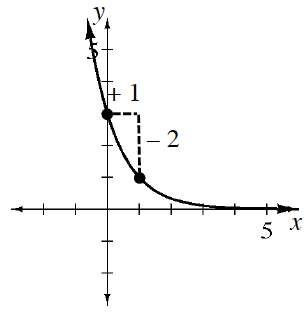### Home > GB8I > Chapter 9 Unit 10 > Lesson INT1: 9.1.2 > Problem9-20

9-20.

Use the graph at right to write an equation in $y = ab^x$ form.

The $a$ value is the initial value or $y$-intercept.

The $b$ value is the multiplier. Think of the two given points in a table like you used in finding geometric sequences. What number would you multiply $3$ by to get $1$?